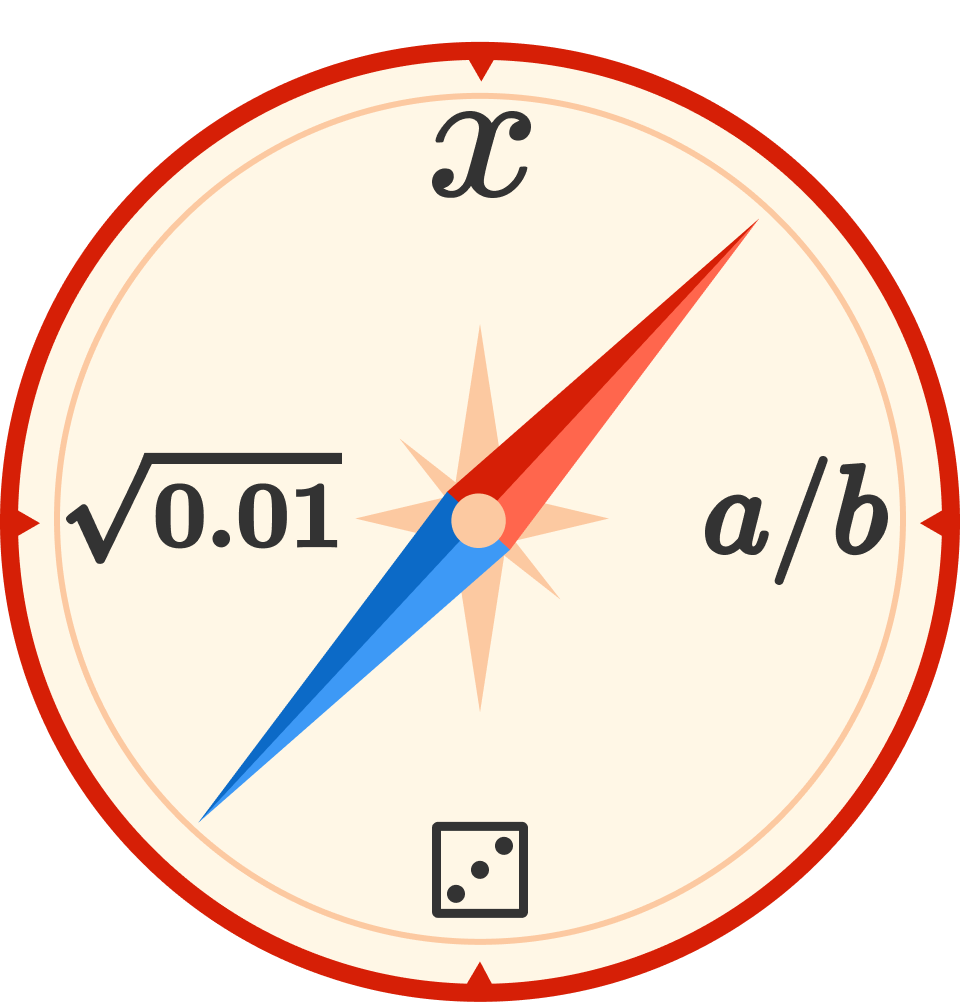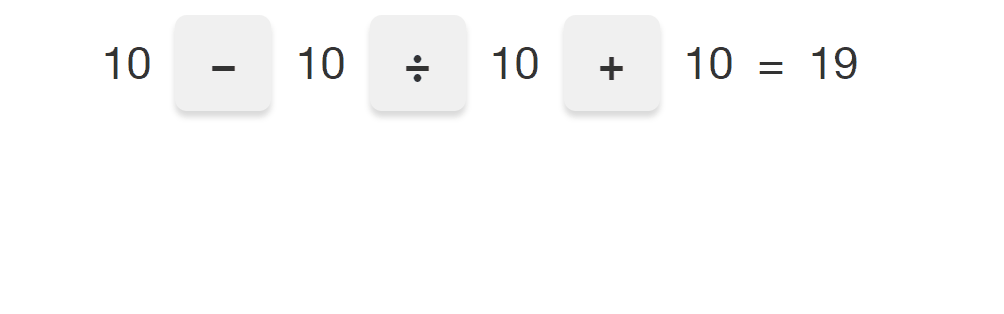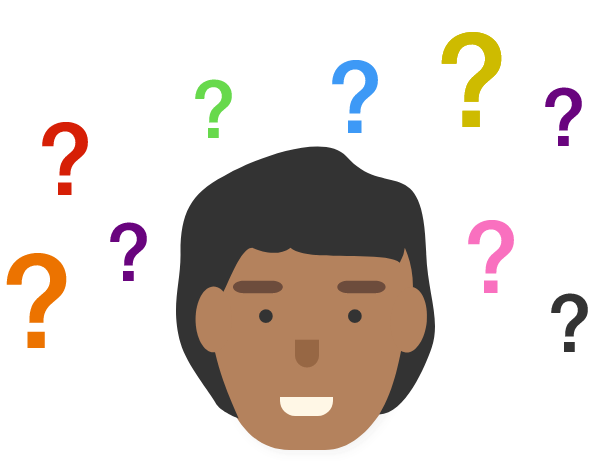# Is It Possible?

In this series of problems, we want to know "is it possible?"

When there are gray boxes in a problem, you can use them to manipulate the equation directly. As shown in the animation below, you can click on one of the operations to change it to a different one.# Is It Possible?

Is it possible to fill in each square with an arithmetic operation $$(+, -, \times, \div)$$ so that the expression equals 101?

# Is It Possible?

$\huge \color{red}A \color{black}\times \color{blue}B \color{black} = \color{green}1000$

If both $$A$$ and $$B$$ are positive whole numbers, is it possible that neither contains the digit 0?

(We are no longer dealing with cryptograms, so $$A$$ and $$B$$ are numbers rather than individual digits.)

# Is It Possible?

Finding examples is one of the most fundamental activities of mathematics, and is useful at every level of difficulty.

Sometimes the examples can be hard to find! If you think something is impossible, try to figure out a logical reason why. It can help to try writing your examples in patterns. You may spot a commonality that must hold true in every case, or you may realize in the process what you're missing.

If a challenge seems impossible, try keeping track of the possible results and listing them out so that you can see the patterns in what's possible vs. what isn't.

# Is It Possible?

Is it possible to make this expression equal 6 using only addition and subtraction signs?

# Is It Possible?

$\Large \frac{\color{blue}{\text{Even}}}{\color{green}{\text{Even}}} \ \times \ \color{purple}{\text{Even}} \ \ \color{black}{=} \ \ \color{red}{\text{Odd}}$

Is it possible for an even number, divided by another even number, times another even number to equal an odd number?

Slow down and think carefully, this might be the hardest question in this sequence!

Hint: is it really true that any number times 2 is even? Can you give a counter-example to this?

# Is It Possible?

$\huge \color{orange}A \color{black}\div \color{purple}B \color{black} = \color{red}1000$

If both $$A$$ and $$B$$ are positive whole numbers, is it possible that neither contains the digit 0? Note the different operation ($$\div$$ instead of $$\times$$).

# Is It Possible?

Generating examples provides us with powerful insights.

If a question asks if something is possible, and we produce an example that meets the conditions, then it's possible.

If a problem asks for something impossible, producing examples of what does work can often indicate a pattern of what doesn't work.

A question may not explicitly ask for what's possible or impossible, yet the possibility or impossibility of something lies at the root of mathematics.In the main course you'll learn more techniques to start conquering every area of mathematics!

×